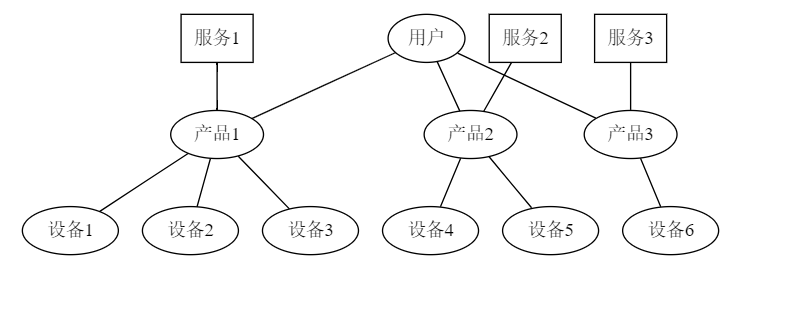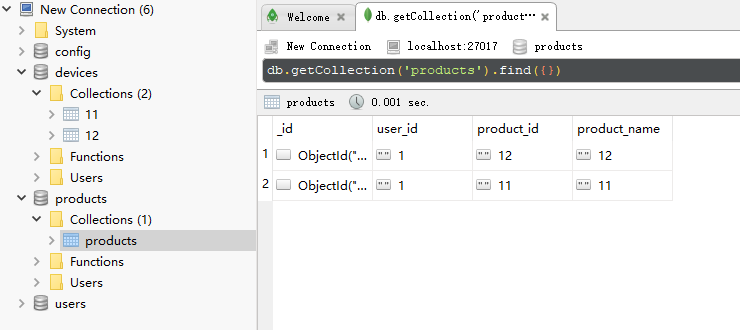# mongodb多数据库查询

### 1. 概述

IoT物联网平台使用了用户-产品-设备模型，进行设备管理。用户、产品、设备分别在不同的mongodb数据库中。其中，设备的表明为产品的ID。### 2. mongodb特性

mongodb支持获取某页数据的函数

1. skip()方法来跳过指定数量的数据
2. limit()方法读取指定数量的数据记录
3. 当limit为0时，获取skip之后的所有数据
db.COLLECTION_NAME.find().limit(NUMBER).skip(NUMBER)


### 3. 实现需求

1. 获取指定页的数据
2. 获取指定数量的数据
3. 当每页数量为0时，则获取全部数据

### 4. Go语言实现

    intPage := int(page)
intSize := int(size)
if intPage < 1 {
intPage = 1
}

devices := []*pb.Device{}
start := (intPage - 1) * intSize // 开始位置(default:0)
end := 0                         // 结束位置(文档末尾)

// 取当前用户的产品
for _, product := range products {
sess := devicess[product.Id]
count := len(sess)
t.Log("count:", count)
end += count
t.Log("start:", start)
t.Log("end:", end)

partDevices := []*pb.Device{}
if end-start <= 0 {
continue
}
// 如果end - start < 需要获取的device
if end-start < intSize {
// 本次需要取的devices数
limit := end - start
// 本次需要跳过的device数
skip := count - (end - start)
// 需要取出的数据减少
intSize -= limit
// start位置提前
start += limit
if limit != 0 {
partDevices = sess[skip : skip+limit]
} else {
partDevices = sess[skip:count]
}
devices = append(devices, partDevices...)
}

if end-start >= intSize {
// 本次需要取的devices数
limit := intSize
// 本次需要跳过的device数
skip := 0
// 需要取出的数据减少
intSize -= limit
// start位置提前
start += limit
if limit != 0 {
partDevices = sess[skip : skip+limit]
} else {
partDevices = sess[skip:count]
}

devices = append(devices, partDevices...)
}
if intSize < 0 || (intSize == 0 && size != 0) {
break
}

}


×

Help us with donation